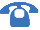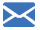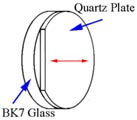CALL US +86 591 2837 9366 +86 591 2837 9336CONTACT US sales@tempotec.com
WaveplatesWaveplates (retardation plates or phase shifters) are made from materials which exhibit birefringence. The velocities of the extraordinary and ordinary rays through the birefringent materials vary inversely with their refractive indices. The difference in velocities gives rise to a phase difference when the two beams recombine. In the case of an incident linearly polarized beam this is given by α=2πd (ne-no) / λ， where α is phase difference; d is thickness of waveplate; ne,no-refractive indices of extraordinary and ordinary rays respectively; λ is wavelength). At any specific wavelength the phase difference is governed by the thickness of the retarder.
 Low-order Waveplate Its properties are much better than the multi-order waveplate because of its thinner thickness (less than 0.5mm). Better temperature (38°C), wavelength (1.5nm) and incident angle (4.5°) bandwidth and high damage threshold make it widely used in common application. Also, it is economical. ...Zero-Order Waveplate It is constructed of two multi-order waveplates with their axis crossed. Thus, it performs as a zero-order waveplate because of the effect of two plates counteracting each other. It has wide temperature bandwidth and wavelength bandwidth properties. Because it is cemented, the damage threshold must...True Zero-Order Waveplate Cemented True Zero-Order Waveplate The true zero-order waveplate means that the thickness of waveplate is very thin (less than 0.1mm) which make the true zero-order waveplate excellent in temperature, wavelength and incident angle (about 20º) bandwidth. Therefore, it is excellent choic...